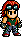# Armor King/Tekken Tag Tournament Movelist

Armor King/Tekken Tag Tournament Moves List is a list of all of the moves that the character Armor King can perform in Tekken Tag Tournament.Looks like Hwoarang has run off and made this page, Armor King/Tekken Tag Tournament Movelist, without telling Baek Doo San and hasn't done it right!Please fix it by re-formatting this page to follow it's [[Template:{{{1}}}|template]].

## §Moves List

1. One Two Combo: Square Triangle.
2. One Two Uppercut: Square Triangle Square.
3. Jab Uppercut: Triangle Square (or East Triangle Square.).
4. Neck Cutter Kick: X + Circle.
5. Toll Kick: West X.
6. Water Parting Chop: West Square.
7. Low Drop Kick: Southwest Circle.
8. Capital Punishment: East Square + Triangle.
9. Frankensteiner: Southeast X + Circle.
10. Corporate Elbow: South Square + Triangle.
11. Double Knee Drop: Northeast X + Circle.
12. Elbow Drop: Northeast Triangle + Circle.
13. Shoulder Tackle: East Square + Circle.
14. Straight Arrow: West Square + Circle.
15. Left Straight Uppercut: South Square (Neutral) Triangle. (Note: This command is wrote as "South Square Triangle" in Armor King's Command List. However, this way doesn't work.).
16. Stagger Kicks: South X + Circle Circle Circle (or: (While crouching) Southeast Circle Circle Circle.).
17. Counter Stagger Kicks: (During a counter hit) South X + Circle Circle Circle Circle Circle (or: (While crouching) Southeast Circle Circle Circle Circle Circle.).
18. K's Flicker: East East (hold) Triangle.
19. Head First Lunge: East East (hold) Square + Triangle.
20. Konvict Kick: East East (hold) Circle.
21. Exploder: East East (hold) X + Circle.
22. Jaguar Thrust: (During sidestep) Triangle.
23. Dynamite Uppercut: (While crouching) Southeast Triangle.
24. Blind Kick: (Back towards enemy) X.
25. . Lifting Elbow: (While rising) Square.
26. Palm Upper: East (Neutral) South Southeast (hold) Triangle.
27. Shadow Lariat: East (Neutral) South Southeast (hold) Square.
28. Jumping Knee: East (Neutral) South southeast (hold) Circle.
29. Stomach Smash: East East (Neutral) Triangle.
30. Burning Knuckle: East Square + Triangle South (hold.).
31. Jumping Moonsault: Northeast (Neutral) Square + Circle.
32. Jumping Stagger Kick: Northeast (Neutral) X + Circle Circle Circle.
33. Jumping Capital Punishment: Northeast (Neutral) Square + Triangle.
34. Jumping Burning Knuckle: Northeast (Neutral) Square + Triangle South (hold.).
35. Running Exploder: East East East X + Circle.
36. Supercharger: X + O + Triangle + Square.
37. 10 Hit Combo: Square Triangle Square Square Triangle Circle Circle Circle Square X.
38. Knee Bash: (Approach enemy) Square + X.
39. Suplex: (Approach enemy) Triangle + Circle.
40. Reverse Death Valley Bomb: Square + X (or Triangle + Circle) (From the left.).
41. Heavy Fall Reverse DDT: Square + X (or Triangle + Circle) (From the right.).
42. Reverse DDT: Square + X (or Triangle + Circle) (From behind.).
43. Reverse Throw: (Back towards enemy) Triangle + Circle.
44. Ultimate Tackle: South (hold) Square + Triangle.
45. Ultimate Punch: (During Tackle) Triangle Square Triangle Square Triangle.
46. Mount Position: (While the enemy is down) Southwest Square + X (or Triangle + Circle.).
47. Jaguar Driver: (Approach enemy) South Southeast East (hold) Square.
48. Jaguar Screwdriver: (Approach enemy) Triangle + Circle South South South (hold) Square + Triangle.
49. Tombstone Piledriver: (Approach enemy) Southwest East (hold) Triangle.
50. Armor King Driver: (Approach enemy while you are crouching) South (hold) Southwest Triangle + Circle.
51. DDT: (Approach enemy) Southwest Southwest (hold) Square + Triangle.
52. Giant Swing: (Approach enemy) East West Southwest South Southeast East (hold) Square. (or: (Approach enemy) East Northeast North Northwest West Southwest South Southeast East (hold) Square.).

CHOKE SLEEPER COMBO.

1. Choke Sleeper: (Approach enemy) East (Neutral) South Southeast (hold) Square + Triangle.
2. Half Nelson Press: (During Choke Sleeper) Triangle + Circle Square + Triangle Square + Triangle.
3. Falling Sleeper Chain Throw: (During Choke Sleeper) X + Circle X + Circle Square + Triangle.
4. Triple Mount Punches: (During Falling Sleeper Chain Throw) Square Square Square + Triangle.
5. Stretch Muffler: (During Falling Sleeper Chain Throw) X Circle Square + Triangle X + Circle.

1. One Two Uppercut to Elbow Sting: Square Triangle Square Square.
2. One Two Uppercut to Elbow Sting to Smashing Hook: Square Triangle Square Square Triangle.
3. Jab Uppercut to Elbow Sting: East Triangle Square Square.
4. Jab Uppercut to Elbow Sting to Smashing Hook: East Triangle Square Square Triangle.
5. Elbow Sting: Southeast Square.
6. Smashing Hook: Southeast Triangle.
7. Taurus Cutter: South X + Circle (or: (While crouching) Southeast Circle.).
8. Jumping Taurus Cutter: Northeast (Neutral) X + Circle.
9. 9 Hit Combo: East Triangle Square Square Triangle Circle Circle Circle Square X.
10. Rising Uppercut: (While rising) Triangle.
11. Ultimate Punch 2: (During Tackle) Square Triangle Square Triangle Square.